StatLect

# Design matrix

A design matrix is a matrix containing data about multiple characteristics of several individuals or objects. Each row corresponds to an individual and each column to a characteristic.

The design matrix is a fundamental mathematical object in regression analysis, for example, in linear regression models and in logit models. It is often denoted by the capital letter.## Examples

We provide here some examples of design matrices.

Example If we measure the height and weight of five individuals, we can collect the measurements in a design matrix having five rows and two columns. Each row corresponds to one of the ten individuals, the first column contains the height measurements and the second one reports the weights: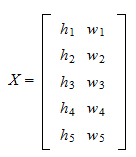wheredenotes the height of the-th individual andher weight.

Example If we collect the data about the gross domestic product (GDP) of four countries in three consecutive years, then the design matrix is thematrixwhere, for example,is the GDP of the third country in the second year.

## How the design matrix is defined in linear regressions

Consider the linear regression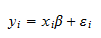whereis the dependent variable,is avector containing theexplanatory variables (regressors),is a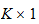vector of regression coefficients,is the error term and there areobservations (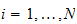).

Thus, we observecharacteristics, contained in the vector of regressors, for each of theobservations.

All the observations can be collected in the design matrix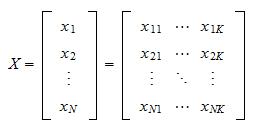wheredenotes the-th entry of the vector, that is, the-th regressor.

We can similarly stack the observations of the dependent variable and the error terms into twovectors: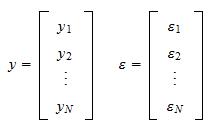Having defined the design matrixand the two vectorsand, we can write the regression equations in matrix form:This allows us to use matrix algebra to find an estimator of the regression coefficients(see the lecture on linear regression to see how).

## Rank of the design matrix

In most statistical models the design matrix is required to have full-rank, that is, its columns must be linearly independent (see, e.g., the normal linear regression model). When this requirement is not met, we say that the design matrix suffers from multicollinearity (see this lecture for details).

However, there are also regression models where the design matrix can be rank-deficient (i.e., not full-rank), for example the Ridge regression model.

## More details

See the lecture on linear regression models for more details.

## Keep reading the glossary

Previous entry: Cross-covariance matrix

Next entry: Discrete random variable